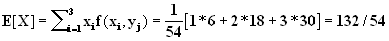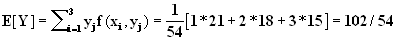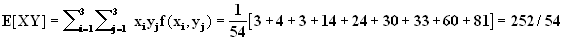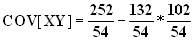LEGACY CONTENT. If you are looking for Voteview.com, PLEASE CLICK HERE

This site is an archived version of Voteview.com archived from University of Georgia on May 23, 2017. This point-in-time capture includes all files publicly linked on Voteview.com at that time. We provide access to this content as a service to ensure that past users of Voteview.com have access to historical files. This content will remain online until at least January 1st, 2018. UCLA provides no warranty or guarantee of access to these files.

Probability and Statistics                      Name__________________________
Spring 1997 Flex-Mode 45-733
Midterm
Keith Poole

(10 Points)

1. You draw 12 cards randomly without replacement from a deck of 52 playing cards. What is the probability that you get 5 of one suit, 4 of a second suit, 2 of a third suit, and 1 of fourth suit (e.g., © © © © © ¨ ¨ ¨ ¨ § § ª ).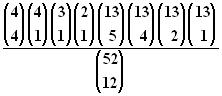Probability and Statistics                      Name__________________________
Spring 1997 Flex-Mode 45-733
Midterm
Keith Poole

(10 Points)

2. Suppose we have the continuous probability distribution

f(x) =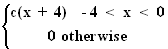a. Find c.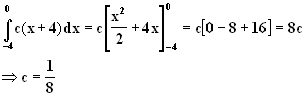b. Find F(x).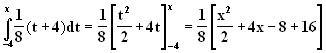F(x) =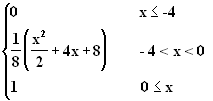Probability and Statistics                      Name__________________________
Spring 1997 Flex-Mode 45-733
Midterm
Keith Poole

(10 Points)

3. Consider the following portion of an electric circuit with ten relays. Current will flow from point A to point B if there is at least one complete path when the relays are activated. The relays are turned on simultaneously and their failures are independent of one another. The relays are identical in every respect and the probability that a relay fails is 0.1. What is the probability that current will flow from A to B when the relays are activated?
```
|--1-----2--|   |--6----------7--|
|           |   |                |
A--|-----3-----|---|                |--B
|           |   |     |-- 9--|   |
|--4-----5--|   |--8--|      |---|
|--10--|
```
P[current flows from A to mid point] = 1 - P[all three parts fail] = 1 - (0.19*0.1*0.19)
P[current flows from mid point to B] = 1 - P[both parts fail] = 1 - (0.19*(0.1+0.01-0.1*0.01))

So, P[current flows from A to B] = [1 - (0.19*0.1*0.19)][1-(0.19*0.109)] = 0.9758

Probability and Statistics                      Name__________________________
Spring 1997 Flex-Mode 45-733
Midterm
Keith Poole

(10 Points)

4. Approximately 20% of boxes coming off an assembly line have serious flaws. If ten boxes are randomly selected, find the probability distribution for the number of defective boxes in the sample and calculate the mean and variance of this distribution. If at least 5 boxes are defective, what is the probability that at least 6 are defective?

Binomial, n = 10, p = 0.2
Mean = np = 2
VAR = np(1-p) = 8/5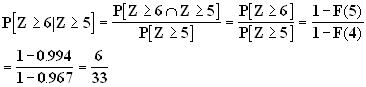Probability and Statistics                      Name__________________________
Spring 1997 Flex-Mode 45-733
Midterm
Keith Poole

(10 Points)

5. Suppose we have a discrete bivariate probability distribution

f(x,y) =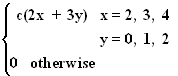a. Find c.

```
y
0   1   2
------------
2 | 4   7  10 |21
|           |
x  3 | 6   9  12 |27
|           |
4 | 8  11  14 |33
|           |
---------------
18  27  36 |81
```

Hence, c = 1/81

b. Find P(X £ 3 ½ Y £ 1)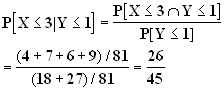Probability and Statistics                      Name__________________________
Spring 1997 Flex-Mode 45-733
Midterm
Keith Poole

(10 Points)

6. Five identical urns are labeled 1, 2, 3, 4, and 5. Urn i contains i white balls, 2i red balls, and 3i green balls (e.g., Urn 3 would contain 3 white, 6 red, and 9 green balls). An urn is randomly selected and two balls are randomly drawn without replacement from the urn. What is the probability that both balls are white.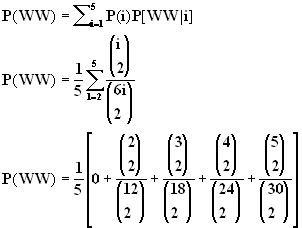Probability and Statistics                      Name__________________________
Spring 1997 Flex-Mode 45-733
Midterm
Keith Poole

(10 Points)

7. Based on many geological surveys, an energy company has classified geological formations beneath potential oil wells as types I, II, and III. For a particular site in which the company is considering drilling for oil, the probabilities of 0.40, 0.45, and 0.15 are assigned to the three types of formations respectively. It is known from experience that oil is discovered in 35 percent of type I formations, in 25 percent of type II formations, and 20 percent of type III formations. If oil is discovered at this site, determine the probability that a type II formation exists.

O = "0il"
P(I) = 0.4,      P(O|I) = 0.35
P(II) = 0.45,      P(O|II) = 0.25
P(III) = 0.15,      P(O|III) = 0.2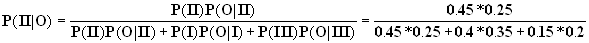Probability and Statistics                      Name__________________________
Spring 1997 Flex-Mode 45-733
Midterm
Keith Poole

(10 Points)

8. Suppose we have the bivariate continuous probability function

f(x,y) =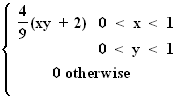a) Find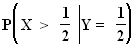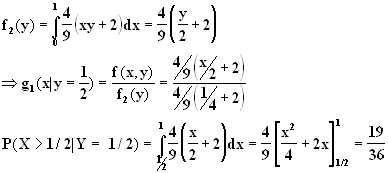b) Find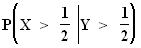P(X > 1/2|Y > 1/2) =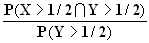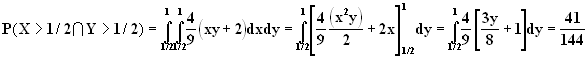By symmetry from (a),

P(Y > 1/2) = 19/36

Therefore,

P(X > 1/2|Y > 1/2) = 41/76

Probability and Statistics                      Name__________________________
Spring 1997 Flex-Mode 45-733
Midterm
Keith Poole

(10 Points)

9. Supposed we have the probability distribution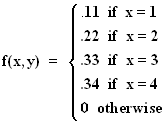a. What is E(X).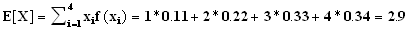b. What is VAR(X).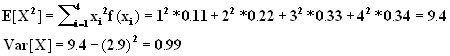Probability and Statistics                      Name__________________________
Spring 1997 Flex-Mode 45-733
Midterm
Keith Poole

(10 Points)

10. Suppose we have a discrete bivariate probability distribution

f(x,y) =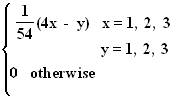Find COV(X, Y).

```
y
1   2   3
------------
1 | 3   2   1 | 6
|           |
x  2 | 7   6   5 |18
|           |
3 |11  10   9 |30
|           |
---------------
21  18  15 |54
```